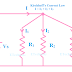Kirchhoff's Current Law(KCL) and Kirchhoff's Voltage Law(KVL) are the two very important laws for analyzing a simple electrical circuit. It gives you a basic idea of how current and voltage react in an electrical circuit. Gustav Robert Kirchhoff, a german physicist discovered these two fundamental rules to better understand the circuit theory. Remember that KCL and KVL can be applied to the both AC and DC circuits but their calculation may slightly different.

## Kirchoff's Current Law(KCL)

KCL states that the algebraic sum of all the incoming and outgoing currents at a node of an electric circuit is Zero. That means the total current entering a node is exactly equal to the total current leaving that node. For example, if a 2A current enters a node or junction, the exact 2A current also leaves the junction.

• KCL is based on the conservation of electric charge
• KCL only applies if the circuit is closed because in an open circuit current cannot flow.
• KCL does not apply to distributed networks, here very difficult to measure current flow to so many directions.
• KCL can not be implemented properly in high-frequency AC circuits.
• KCL applies very properly with resistive circuits, it is difficult for other circuits such as capacitors, inductors, and other active components. This is because those components are influenced by different parameters such as current flow, frequency, magnetic field, etc.
• It is very easy to calculate if the current flow is constant.
• The current flow should not be affected by external factors.

### KCL Examples and Application

• Just take an example, two LEDs are connected in series. And the connection between two LEDs is a node. If those two LEDs have different ratings then also the current flow in the whole circuit will be the same as it is a series circuit. Now, the node between the two LEDs will have zero current as the incoming and outgoing currents are equal.
• Now, if we assume that two different values of LEDs are connected in parallel. The connection of two LEDs and the power supply can be taken as a node or junction. The incoming current comes to the node from the power supply and the outgoing current from the node goes to those two LEDs. For example, if those two LEDs take current 2mA and 3mA respectively. So the total outgoing current from the node is 2mA+3mA = 5mA. Now the current flow from the power source to that note will 5mA. So, you may understand each led and power source is involved in different current flows but at the node, incoming and outgoing current flow is the same.
• So DC parallel circuits consisting of resistors are able to analyze with KCL law.
• KCL law is used to measure and calculate the current flow in a circuit.
• Wheatstone bridge is an example of KCL.

## Kirchoff's Voltage Law(KVL)

KVL states that the algebraic sum of voltage drops in any closed path or circuit is equal to the Source voltage. Or in other words, we can say, the algebraic sum of all the voltages in a closed circuit is zero with respect to a specific direction. KVL helps us to analyze the voltage distribution in a circuit.

Some important notes about KVL Law,
• It is also based on the conservation of electric charge.
• KVL is not applied to distributed networks.
• KVL is not possible to calculate if there is no current flow in the circuit or the circuit is fully short-circuited where we cannot get the resistance value.
• A magnetic field in a closed circuit can badly affect the calculation of KVL.
• In an open circuit, we can get the voltage across the power source only, we cannot get voltage across other elements.

### KVL Examples and Application

• Let's take an example, two LEDs are connected in series with a 5V power supply. When the power supply is turned ON, the voltage drop across those LEDs will be that the sum of voltage drop across those LEDs will be equal to the supply voltage 5V. It may be 4V and 1V or 3V and 2V or 2.5V and 2.5V. So the total voltage drop always is equal to the 5V.
• So DC series circuits with resistive elements can be taken as examples for the KVL calculation.
• KVL is used to measure the voltage distribution in an electric circuit.
• KVL and KCL both are helpful for finding unknown resistance in a circuit.
• Wheatstone bridge is a great example of KVL.

KCL and KVL Real Applications, Examples | Kirchhoff's LawReviewed by Author on October 25, 2022 Rating: 5